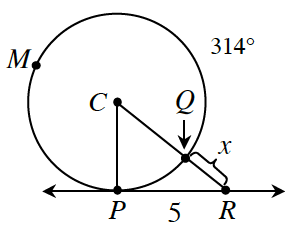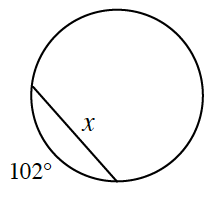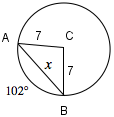### Home > CCG > Chapter 12 > Lesson 12.2.3 > Problem12-93

12-93.

Examine the diagrams below. For each one, use the geometric relationships to solve for the given variable.

1. $\overleftrightarrow{PR}$ is tangent to $\odot C$ at $P$ and $m \overarc{ P M Q } = 314^\circ$. Find $QR$.$\angle PCQ = 360 - 314 = 46^\circ$

$\text{tan}46^\circ = \frac{5}{\overline{CP}}$

$\overline{CP} = \overline{CQ} \approx 4.83$

$\text{sin}46^\circ = \frac{5}{\overline{CR}}$

$\overline{CR} \approx 6.95$

$x\approx6.95-4.83\approx2.12$

1. Radius = $7$ cmDraw $\Delta ABC$ out of the chord that has been given.According to the Law of Cosines:

$\overline{AB}^2 = \overline{AC}^2 + \overline{BC}^2 - 2 (\overline{AC}) (\overline{BC}) \text{cos}102^\circ$

$x^2 = 7^2 + 7^2 - 2(7)(7)\text{cos}102^\circ$